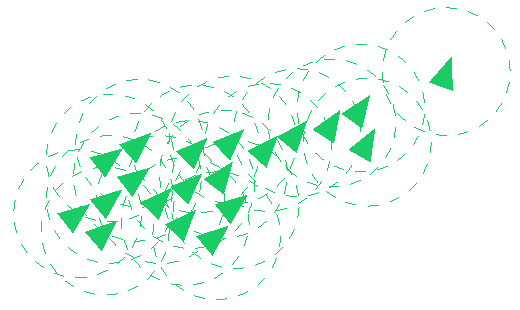# CPSC170AFundamentals of Computer Science II

## Project 4

BoidsSimulating the flocking of birds, the schooling of fish, the swarming of insects, or the herding of animals can be achieved with just a few simple rules. Each agent in the simulation, called boids, steers according to the the other boids that are close to it, its neighborhood. Different rules can be used some common ones are:

1. Separation: boids want space between them and neighbors
2. Alignment: boids want to move in the same direction as neighbors
3. Cohesion: boids want to be near their neighbors
4. Goal: boids want to move to a goal
5. Inertia: boids want to keep moving in the same direction

Each of these rules can be turned into a direction to move with the following equations:

$$\vec{s} = \frac{\sum_{j=1}^{n} \vec{p_j} - \vec{p}}{-n}$$

$$\vec{a} = \frac{\sum_{j=1}^{n} \vec{d_j}}{n}$$

$$\vec{c} = \frac{\sum_{j=1}^{n} \vec{p_j}}{n} - \vec{p}$$

$$\vec{g} = \vec{l} - \vec{p}$$

$$\vec{i} = \vec{d}$$

Where $\vec{s}$, $\vec{a}$, $\vec{c}$, $\vec{g}$, and $\vec{i}$ are the directions for a boid to move produced by the separation, alignment, cohesion, and goal rules. The vectors $\vec{p}$ and $\vec{d}$ are the position and direction of movement of a boid, $\vec{p_j}$ and $\vec{d_j}$ are the position and direction of the jth boid in a boid’s neighborhood, and $\vec{l}$ is the location of the boid’s goal.

Often these different rules are in conflict. To combine the direction produced by all of the rules they are normalized and weighted, so that some are more important and others are less important, and summed together to produce one direction using the equation:

$$\vec{d'} = \hat{s}w_s + \hat{a}w_a + \hat{c}w_c + \hat{g}w_g + \hat{i}w_i$$

Where $\vec{d'}$ is the boid’s new direction of movement and ws, wa, wc, wg, and wi are the weights of each of the separation, alignment, cohesion, goal, and inertia rules. The larger a weight is, the more it contributes to the boids direction of movement in comparison to the other weights. If there are no other boids in a boid’s neighborhood, then the separation, alignment, and cohesion weights should be set to zero when computing the new direction. Different weights will produce different flocking behaviors. For example, increasing the cohesion weight will decrease the distance between boids and shrink the overall size of the flock.

The new position of a boid can be calculated from the direction using the following equation:

$$\vec{p'} = \hat{d'}(r * t) + \vec{p}$$

Where $\vec{p'}$ is the boid’s new position, $\vec{p}$ is the boid’s current position, r is the boid’s rate of movement, or speed, in units per second, and t is the amount of time that has elapsed since the boid’s position was last updated, in seconds.

#### Details

Create a program that simulates a two-dimensional flock (or school or swarm or herd) using the boids algorithm. The program should use OpenGL to draw the flock as triangles. The goal of all of the boids in the flock should be the same, the location of the user’s mouse.

The program must use a hash table, implemented as a two-dimensional array of lists, to store all of the boids. The size of the two-dimensional array should correspond to the size of a boid’s neighborhood so that the entirety of a boid’s neighborhood can be found in the nine hash table cells that are nearest to a boid.

Draw a boid as a triangle pointed in the direction of its movement. Draw the same triangle for every boid and use the glRotatef and glTranslatef functions to rotate and translate each to the correct orientation and position. To calculate the rotation angle use the atan2(direction_y, direction_x) function in the cmath library. Note, this function returns the number of degrees in radians of the specified direction vector but the glRotatef function takes an angle in degrees.

### Extra Credit

Boids are used to simulate flocking behavior in video games and movies, but they can also be used for more practical purposes. They can be used to model the movements of people. This can be useful for testing architectural designs to improve safety during evacuations. To model people in a building, the boids will also need to avoid obstacle. Add obstacle to the simulation, triangles that do not move, that the boids will move away from if they get too close.

### Collaboration

You may collaborate with a partner on this assignment. If you do, both partners must work on the same computer and actively contribute to every line of code. Only one partner needs to submit code, but both partners names should be in the code.

### Grading

The project will be graded according to the following weights:

20% - Style, design, and documentation

20% - Test cases (and assertions)

10% - Has a hash table implemented as a two-dimensional array of lists

10% - Draws boids as triangles

10% - Updates boids’ position every frame of animation

10% - Updates boids’ position using rules

10% - Boids move toward the mouse

10% - Boids demonstrate flocking behavior

### Submission

Submit all file for your program on the course Inquire page before class on Monday, April 30th.